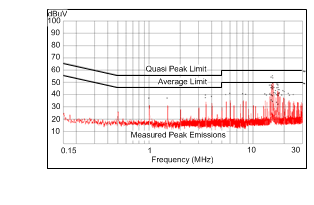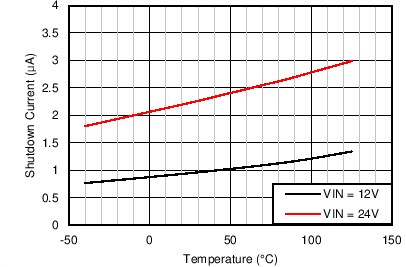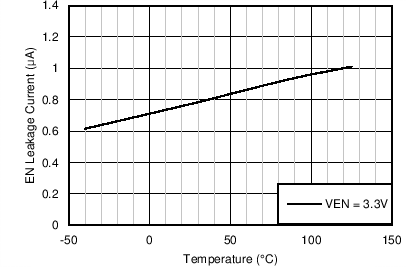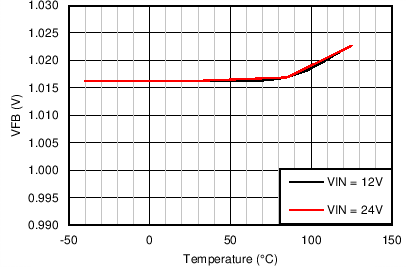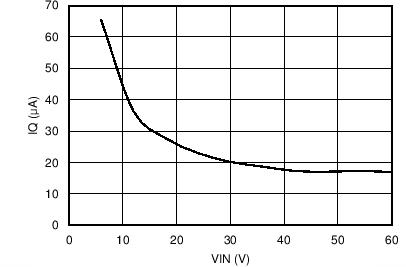SNVSA46B June   2014  – January 2018

PRODUCTION DATA.

1. Features
2. Applications
3. Description
1.     Device Images
4. Revision History
5. Pin Configuration and Functions
6. Specifications
7. Detailed Description
1. 7.1 Overview
2. 7.2 Functional Block Diagram
3. 7.3 Feature Description
4. 7.4 Device Functional Modes
8. Applications and Implementation
1. 8.1 Application Information
2. 8.2 Typical Applications
1. 8.2.1 Design Requirements
2. 8.2.2 Detailed Design Procedure
3. 8.2.3 Application Curves
9. Power Supply Recommendations
10. 10Layout
1. 10.1 Layout Guidelines
2. 10.2 Layout Example
11. 11Device and Documentation Support
12. 12Mechanical, Packaging, and Orderable Information

• PWP|16
• PWP|16

### 6.8 Typical Characteristics

Unless otherwise specified, VIN = 24 V, VOUT = 3.3 V, FS = 500 kHz, L = 18 µH, COUT = 100 µF, CFF = 33 pF. See Application Curves for bill of materials (BOM) for other VOUT and FS combinations.VOUT = 3.3 V FS = 500 kHz
Figure 1. Efficiency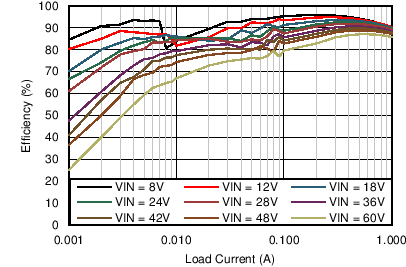VOUT = 5 V FS = 200 kHz
Figure 3. Efficiency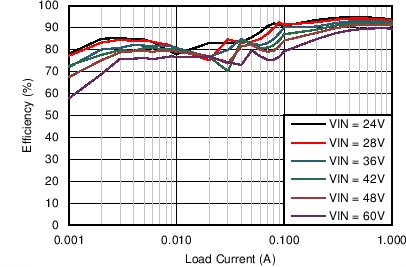VOUT = 12 V FS = 500 kHz
Figure 5. Efficiency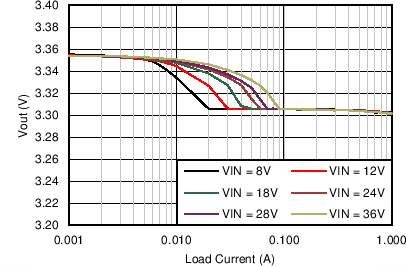VOUT = 3.3 V FS = 500 kHz
Figure 7. VOUT Regulation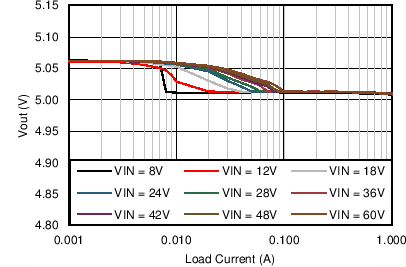VOUT = 5 V FS = 200 kHz
Figure 9. VOUT Regulation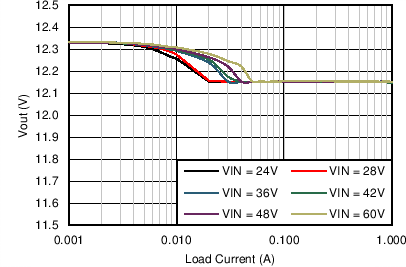VOUT = 12 V FS = 500 kHz
Figure 11. VOUT Regulation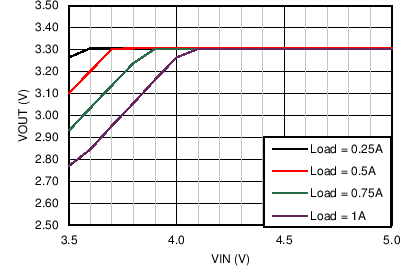VOUT = 3.3 V FS = 500 kHz
Figure 13. Dropout Curve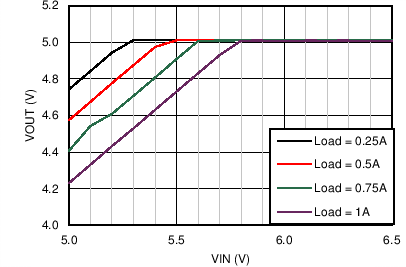VOUT = 5 V FS = 200 kHz
Figure 15. Dropout CurveVOUT = 12 V FS = 500 kHz
Figure 17. Dropout Curve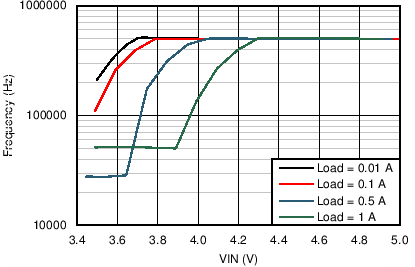VOUT = 3.3 V FS = 500 kHz
Figure 19. Switching Frequency vs VIN in Dropout Operation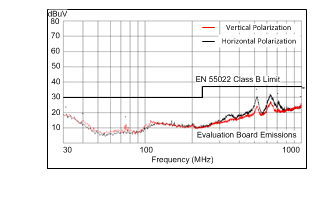VOUT = 3.3 V FS = 500 kHz IOUT = 1 A Measured on the LM46001PWPEVM with default BOM. No input filter used.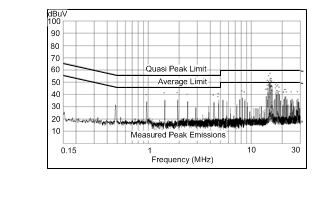VOUT = 3.3 V FS = 500 kHz IOUT = 1 A Measured on LM46001PWPEVM with default BOM. EVM input filter: Lin = 1 µH Cd = 47 µF CIN4 = 68 µF
Figure 23. Conducted EMI Curve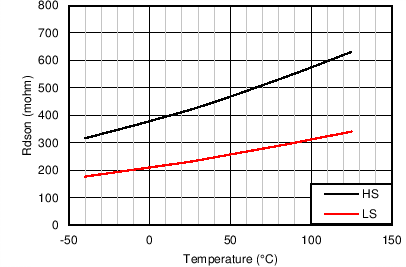Figure 25. High-Side and Low-side On Resistance vs Junction Temperature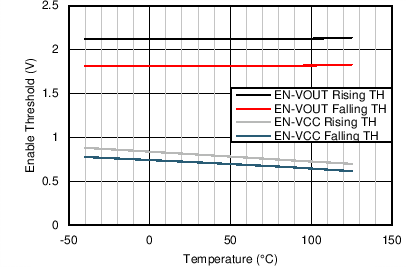Figure 27. Enable Threshold vs Junction Temperature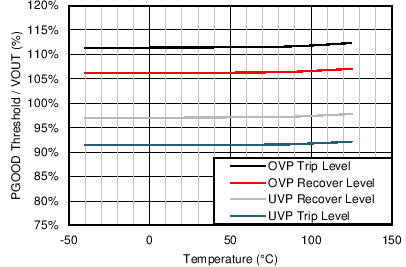Figure 29. PGOOD Threshold vs Junction Temperature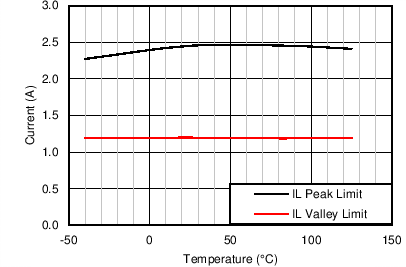VIN = 24 V VOUT = 3.3 V FS = 500 kHz
Figure 31. Peak and Valley Current Limits vs Junction Temperature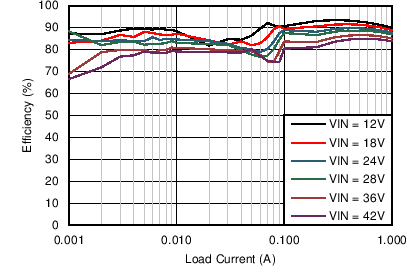VOUT = 5 V FS = 500 kHz
Figure 2. Efficiency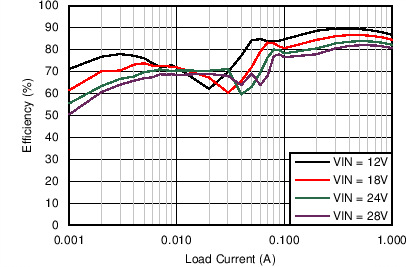VOUT = 5 V FS = 1 MHz
Figure 4. Efficiency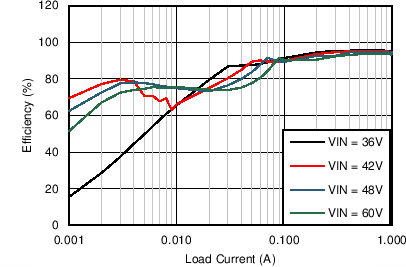VOUT = 24 V FS = 500 kHz
Figure 6. Efficiency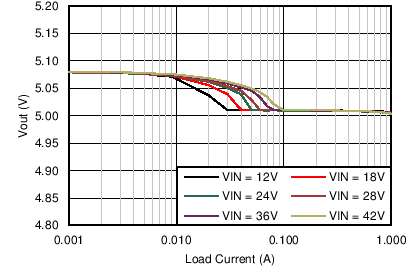VOUT = 5 V FS = 500 kHz
Figure 8. VOUT Regulation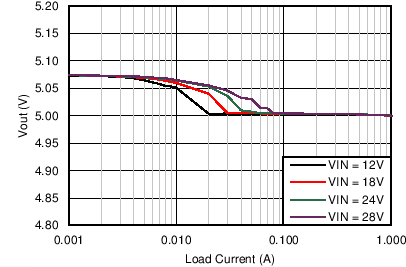VOUT = 5 V FS = 1 MHz
Figure 10. VOUT Regulation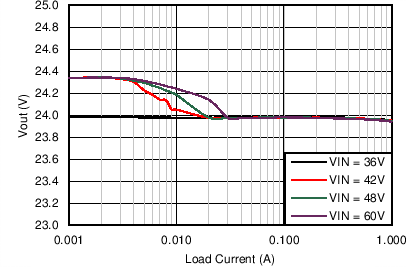VOUT = 24 V FS = 500 kHz
Figure 12. VOUT Regulation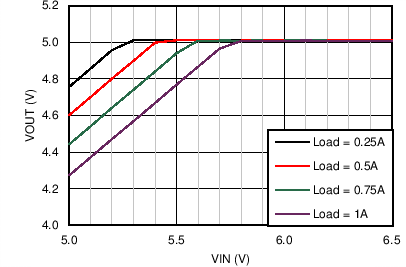VOUT = 5 V FS = 500 kHz
Figure 14. Dropout Curve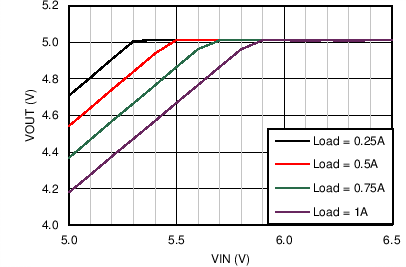VOUT = 5 V FS = 1 MHz
Figure 16. Dropout CurveVOUT = 24 V FS = 500 kHz
Figure 18. Dropout CurveVOUT = 5 V FS = 1 MHz
Figure 20. Switching Frequency vs VIN in Dropout Operation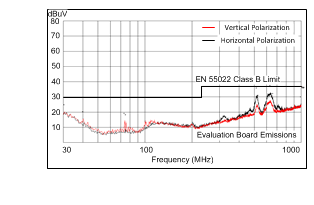VOUT = 5 V FS = 500 kHz IOUT = 1 A Measured on the LM46001PWPEVM with L = 27 µH, COUT = 66 µF, CFF = 33 pF. No input filter used.